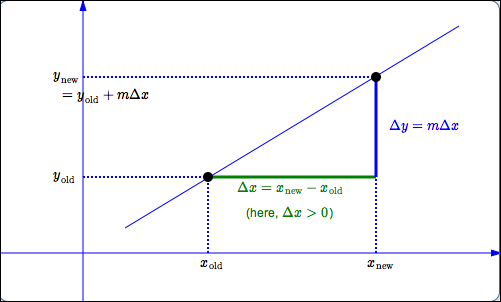﻿ Working with Linear Functions

# WORKING WITH LINEAR FUNCTIONS: FINDING A NEW POINT, GIVEN A POINT AND A SLOPE

• PRACTICE (online exercises and printable worksheets)

Suggested review of lines from the Algebra I curriculum:

Recall:
$$\text{slope of line through points } (x_1,y_1) \text{ and } (x_2,y_2) \ \ =\ \ m \ \ =\ \ \frac{\text{rise}}{\text{run}} \ \ =\ \ \frac{\text{change in } y}{\text{change in } x}\ \ =\ \ \frac{\Delta y}{\Delta x} \ \ =\ \ \frac{y_2 - y_1}{x_2 - x_1}$$
A common scenario is (see diagram at right):

 you have a line with known slope $\,m\,$ you know the coordinates of one point on the line; call this known point $(x_{\text{old}},y_{\text{old}})$ there is another point on the line whose coordinates are needed; call this desired point $(x_{\text{new}},y_{\text{new}})$ you know the change in $\,x\,$ between the old and new point: $$\Delta x = x_{\text{new}} - x_{\text{old}}$$ \begin{alignat}{2} \Delta x > 0\,\ \ &\iff\ \ &&\text{the new point lies to the right of the old point}\cr \Delta x < 0\,\ \ &\iff\ \ &&\text{the new point lies to the left of the old point} \end{alignat} you want the $y$-coordinate, $\,y_{\text{new}}\,$, of the new pointSolving for $\,y_{\text{new}}\,$ in terms of the known quantities:

$\displaystyle m = \frac{y_{\text{new}} - y_{\text{old}}}{x_{\text{new}} - x_{\text{old}}}$

$\Rightarrow\ \ y_{\text{new}} - y_{\text{old}} = m \overbrace{(x_{\text{new}} - x_{\text{old}})}^{\Delta x}$

$\Rightarrow\ \ y_{\text{new}} = y_{\text{old}} + m\Delta x$

EXAMPLE:

You have a known point $\,(1,-5)\,$ on a line with slope $\,7.5\,$.
When $\,x = 1.4\,$, what is the $y$-value of the point on the line?

SOLUTION:

The change in $\,x\,$ in going from the known point ($x = 1$) to the new point ($x = 1.4$) is:   $\,\Delta x = 1.4 - 1 = 0.4\,$

$y_{\text{new}} = y_{\text{old}} + m\Delta x = -5 + (7.5)(0.4) = -2$
Master the ideas from this section